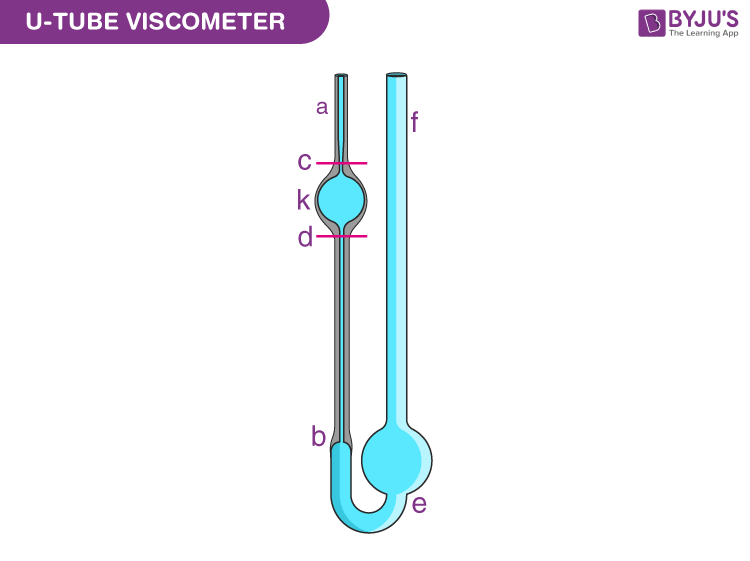# Viscosity

In this article, you will learn what viscosity is, how it is measured, and the viscosity equation of a fluid. You’ll also explore the reasons why viscosity is important.

## What is Viscosity?

Most fluids offer some resistance to motion, and we call this resistance “viscosity.” Viscosity arises when there is relative motion between layers of the fluid. More precisely, it measures resistance to flow arising due to the internal friction between the fluid layers as they slip past one another when fluid flows. Viscosity can also be thought of as a measure of a fluid’s thickness or its resistance to objects passing through it.

A fluid with large viscosity resists motion because its strong intermolecular forces give it a lot of internal friction, resisting the movement of layers past one another. On the contrary, a fluid with low viscosity flows easily because its molecular makeup results in very little friction when it is in motion. Gases also exhibit viscosity, but it is harder to notice in ordinary circumstances.

### Viscosity Definition

The definition of viscosity is as follows:

Viscosity is a measure of a fluid’s resistance to flow.

The SI unit of viscosity is poiseiulle (PI). Its other units are newton-second per square metre (N s m-2) or pascal-second (Pa s.) The dimensional formula of viscosity is [ML-1T-1].

The viscosity of liquids decreases rapidly with an increase in temperature, and the viscosity of gases increases with an increase in temperature. Thus, upon heating, liquids flow more easily, whereas gases flow more slowly. Also, viscosity does not change as the amount of matter changes, therefore it is an intensive property.

### Why does Water flow faster than Honey?## Viscosity Formula

Viscosity is measured in terms of a ratio of shearing stress to the velocity gradient in a fluid. If a sphere is dropped into a fluid, the viscosity can be determined using the following formula:

 $$\begin{array}{l}η=\frac{2ga^2(∆ρ)}{9v}\end{array}$$

Where ∆ρ is the density difference between fluid and sphere tested, a is the radius of the sphere, g is the acceleration due to gravity and v is the velocity of the sphere.

## Viscosity Types

Viscosity is the measure of fluid’s friction to its flow. There are two ways to measure the fluid’s viscosity as follows:

• Dynamic Viscosity (Absolute Viscosity)
• Kinematic Viscosity

One way is to measure the fluid’s resistance to flow when an external force is applied. This is known as Dynamic Viscosity. And the other way is to measure the resistive flow of a fluid under the weight of gravity. We call this measure of fluid viscosity kinematic viscosity.

Many are confused between the two viscosity measures and consider them to be one and the same. In reality, they have significant differences between them. For a few applications, kinematic viscosity is more useful than absolute or dynamic viscosity.

### Newtonian and Non-Newtonian Fluids

Temperature and pressure affect the viscosity of a fluid. The viscosity of liquids increases rapidly with an increase in temperature. On increasing pressure viscosity of liquid molecules increases due to the increase in the resistance to the flow of liquid.

If the viscosity does not change with pressure, we describe something as being a Newtonian fluid. And, if the viscosity does change as stress or temperature changes, we describe something as being a non-Newtonian fluid. Water is an example of Newtonian fluid and toothpaste is an example of Non-Newtonian fluid.

Read More: Newtonian and Non-Newtonian Fluid

## Viscosity Measurement

The elementary way of measuring viscosity is to allow a sphere, such as a metal ball, to drop through a fluid and time the fall of the metal ball. The slower the sphere falls, the greater the viscosity. But, a more accurate measure of viscosity is given by the viscometer.

### U-Tube Viscometer

U-tube viscometers are also known as glass capillary viscometers or Ostwald viscometers.

A viscometer consists of two reservoir bulbs and a capillary tube. In one arm of the U is the capillary, a vertical section of a precise narrow bore. Above, which is a bulb, and with it is another bulb lower down on the other arm, as shown in the image.In use, the upper bulb draws the liquid by suction, and then the liquid is made to flow down through the capillary into the lower bulb. Two marks (one above and one below the upper bulb) indicate a known volume. The time taken for the liquid to pass between these marks is proportional to the kinematic viscosity.

Most commercial units are provided with a conversion factor. The time taken by the test liquid to flow between two points is measured. By multiplying the time measured by the factor of the viscometer, the kinematic viscosity is obtained.

 Related Articles:

## Frequently Asked Questions – FAQs

Q1

### What is Viscosity?

Viscosity is a measure of a fluid’s resistance to flow.
Q2

### Why is viscosity an intensive property?

Viscosity does not change as the amount of matter changes, therefore it is an intensive property.
Q3

### How does viscosity vary with temperature?

The viscosity of liquids decreases rapidly with an increase in temperature, and the viscosity of gases increases with an increase in temperature.
Q4

### How are viscosity and flow rate related?

As the viscosity increases, the flow rate decreases. The flow rate is inversely proportional to viscosity.
Q5

### What is Kinematic Viscosity?

Kinematic viscosity is a measure of a fluid’s internal resistance to flow under gravitational forces.
Stay tuned to BYJU’S and Fall in Love with Learning!